##### Geometry: 1,001 Practice Problems For Dummies (+ Free Online Practice)A polygon is a geometric figure that has at least three sides. The triangle is the most basic polygon. You will find the following formulas and properties useful when answering questions involving triangle inequalities, right triangles, relationships between the angles and sides of triangles, and interior and exterior angles of polygons.

## All triangles

• The sum of the three interior angles of a triangle is 180°.

• The largest side of a triangle is opposite the largest angle of the triangle.

• The sum of the two shorter sides of a triangle must be greater than the longest side of the triangle.

• The exterior angle of a triangle is equal to the sum of the two nonadjacent interior angles of the triangle.

• The centroid of a triangle divides each median of the triangle into segments with a 2:1 ratio.

## Right triangles

• The Pythagorean theorem states that a2 + b2 = c2, where a and b represent the legs of the right triangle and c represents the hypotenuse.

• When you draw an altitude to the hypotenuse of a right triangle, you form two right triangles that are similar to each other and also similar to the original right triangle. Because these triangles are similar, you can set up the following proportions:

• The altitude to the hypotenuse of a right triangle is the mean proportional between the two segments that the hypotenuse is divided into: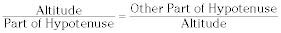• The leg of a right triangle is the mean proportional between the hypotenuse and the projection of the leg on the hypotenuse: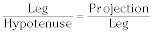•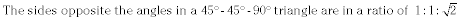•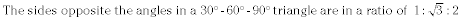• Here are the trigonometric ratios in a right triangle: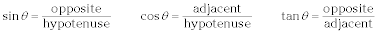## Polygons

• The sum of the degree measure of the interior angles of a polygon equals 180(n – 2), where n represents the number of sides.

• The sum of the exterior angles of a polygon is 360°.

• The area of a regular polygon equals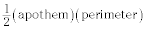The apothem is the line segment from the center of the polygon to the midpoint of one of the sides.